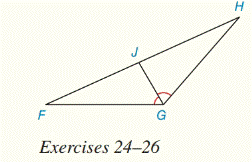Chapter 5.CR, Problem 24CRElementary Geometry For College St...

7th Edition
Alexander + 2 others
ISBN: 9781337614085

Solutions

Chapter
SectionElementary Geometry For College St...

7th Edition
Alexander + 2 others
ISBN: 9781337614085
Textbook Problem

For Review Exercises 24 to 26, G J ¯ bisects ∠ F G HGiven: F G = 10 , G H = 8 , F J = 7 Find JH.

To determine

To find:

JH.

Explanation

Given:

FG=10,GH=8,FJ=7 and

Calculation:

Let JH=x

Then,

Using, “If a ray bisects one angle of a triangle, then it divides the opposite side into segments whose lengths are proportional to the lengths of the two sides that from the bisected angle”.

From, ΔFGH

FGFJ=HGJH

Still sussing out bartleby?

Check out a sample textbook solution.

See a sample solution

The Solution to Your Study Problems

Bartleby provides explanations to thousands of textbook problems written by our experts, many with advanced degrees!

Get Started

Sketch the graph of the equation. 20. |y| = 1

Single Variable Calculus: Early Transcendentals, Volume I

In Exercises 15-18, find the limit (if it exists). limx5x+5x5

Calculus: An Applied Approach (MindTap Course List)

In Exercises 516, evaluate the given quantity. log100,000

Finite Mathematics and Applied Calculus (MindTap Course List)

True or False: x2+1x3dx may be solved using a trigonometric substitution.

Study Guide for Stewart's Single Variable Calculus: Early Transcendentals, 8th